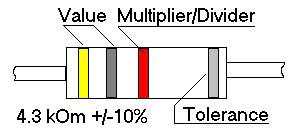References
Physical constants - Electricity - Microscopy and Optics - Unit Conversion - US Drill Size -
Resistor Codes - Capacitor Codes- 2008 Calendar

Useful pH ranges of selected buffers at 25 oC
5.5-7.9

 Buffer MES BIS-TRIS ADA ACES PIPES MOPSO BIS-TRIS PROPANE BES MOPS pKa 6.1 6.5 6.6 6.8 6.8 6.9 6.8; 9.0 7.1 7.2 pH Range 5.5-6.7 5.8-7.2 6.0-7.2 6.1-7.5 6.1-7.5 6.2-7.6 6.3-9.5 6.4-7.8 6.5-7.9

6.8-8.7

 Buffer TES HEPES DIPSO TAPSO TRIZMA HEPPSO POPSO TEA EPPS pKa 7.4 7.5 7.6 7.6 8.1 7.8 7.8 7.8 8.0 pH Range 6.8-8.2 6.8-8.2 7.0-8.2 7.0-8.2 7.0-9.0 7.1-8.5 7.2-8.5 ?? 7.3-8.7

7.4-11.1

 Buffer TRICINE GLYCYL -GLYCINE BICINE TAPS AMPSO CHES CAPSO AMP CAPS pKa 8.1 8.2 8.3 8.4 9.0 9.3 9.6 9.7 10.4 pH Range 7.4-8.8 7.5-8.9 7.6-9.0 7.7-9.1 8.3-9.7 8.6-10.0 8.9-9.3 ?? 9.7-11.1

For more detailed information please refer to Sigma Aldrich Corp.

Major physical constants
c = 2.997925 x 10E8 m/s; h=6.63 x 10E-34 joule*s;   NA =6.023*10E23 mol-1;
g = 9.8 m/s2 ;  ¶ = 3.141;

Kjuri? = 3.7*10E10 s-1.
Electron Charge =1.6*10E-19 coulomb.
Electron Mass  =9.11*10E-28 g.
Proton Mass = 1.67*E-24 g.

Speed of sound in air = 332 m/s; Speed of light = 2997925*10E8 m/s.

Electricity

Resistivity of selected materials - þ - [Ohm*m*10E-8]: Silver - 1.64; Copper - 1.72; Aluminum - 2.83; Nichrom - 100.
Resistance of a conductor with length L and crossection S:
R = þ*L[m]/S[m2]

GHK equation:

E0 = (RT/F)*ln[(PK*Kout + PA*Ain)/(PK*Kin + PA*Aout)]

were: Px - permeability,  Xout , Xin  concentrations inside & outside of ion selective membrane

Microscopy and Optics

Photometric units: 1 candle = 1 lumen/steradian;  1 lux = meter candle = lumen/m2

E = h v = h c/Wavelength;

Refractive Index:  n = sinß1/sinß2
Were ß is an angle between normal to surface and light beam
nair = n vac. = 1; nwater = 1.33; noil = 1.52

Numerical Aperture: NA = n · sinß
Were ß is an angle between objective axis and line connecting focal point to the edge of first lens.

Resolution: d[µm] = Wavelength [µm]/(NAobj+NAcond.)

Useful Magnification:  MU =500-1000 · NAobj

Depth of Focus:
{(1000 · Mobj.) / (7 ·  NAobj.  · MU)} + {(Wavelength[µm]   · MObj2) / (2  · NAObj.2)}

Unit Conversion
Length
1nm =10Å;  1"=25.4 mm; 1' = 30.5 cm;
1 km = 0.62 mile (0.64 sea mile)  1 mile =1.61 km
1yard=91.4 cm
Angle  1 Radian = 180o/¶ = 57.3o

Area 1 acre = 4840 sq. yard = 43560 sq. feet = 4047 sq. meter = 0.405 gectare

Volume
1 liter = 61.02 cubic inch = 0.03531 ft3 = 0.264 gallon = 1.06 quart = 33.81fluid ounce;  1 quart = 0.946 liter.

Mass
1 kg = 1000g = 2.2 lb;
454 gramm (g) = 1 pound (lb) = 16 ounces; 1ounce (oz) = 28g

Temperature/Energy:
oC = (oF - 32)/1.8 = oK-273.
1cal=4.18joule = 0.003968 BTU = 3.968e-8 term
1 Joule = 10E7 erg.

Pressure
1 atmosphere (techn.) = 0.968 atm (phys.) = 1 kg/sm2 = 0.9807 bar = 98070 pascal = 14.22 psi = 735.6 cm of Hg

1 Tesla = 10000 Gauss = 7.96x 10e5 A/m

Technical
US Drill Size number/Diameter in mm.
97         92     83     78       73      67     60     53     47      39     31      21     9
0.15     0.2     0.3     0.4     0.6     0.8    1.0    1.5    2.0     2.5    3.0     4.0     5.0

B      J       O        T       X
6.0   7.0     8.0     9.1     10.1

Resistor Color CodesCOLOR Black Brown Red Orange Yellow Green Blue Violet Gray White DIGIT 0 1 2 3 4 5 6 7 8 9 Multiplier x1 x10 x100 x1K x10K x100K x1M -- -- --
Divider: Silver =x 0.01; Gold = x0.1
Tolerance: None = 20%; Silver=10%; Gold =5%.

Small Capacitor Codes
First two digits  Value in pico-Farads (1 pF=10e-12 F)
Third digit  multiplier [1=10; 2=100; 3=1000; 4=10000; 5=100000]
Letter  tolerance [D=+/- 0.5%; F=1%; G=2%; H=3%; K=10%; M=20%; P=+100%,-0% ; Z=+80%, -20%]
Examples:  47= 47pF; 683=68000pF;  102K=1000pF +/- 10%.